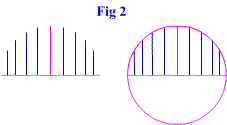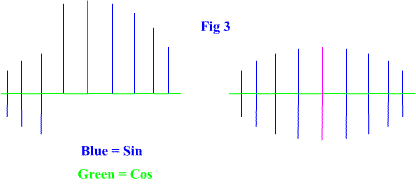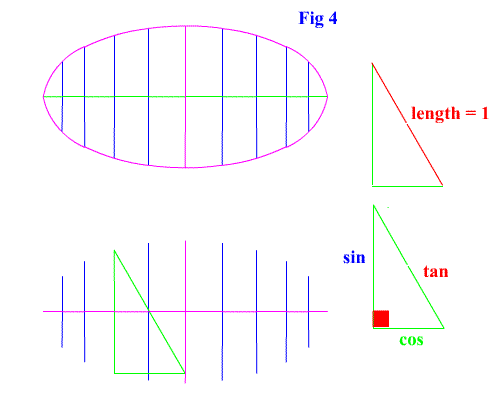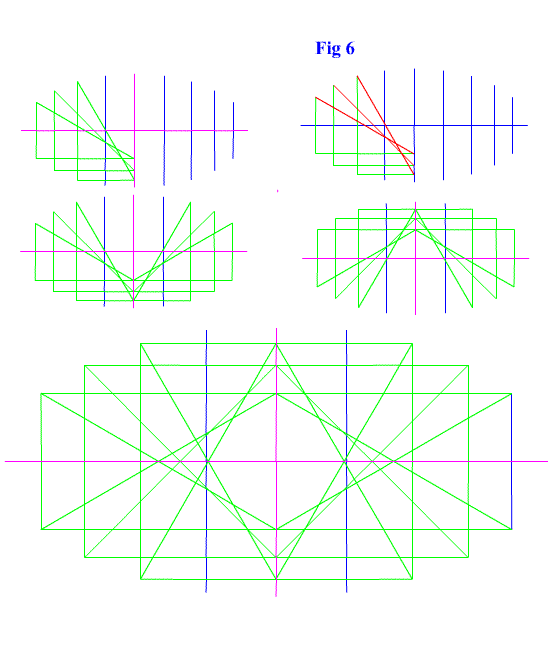\
 <...Previous...> <...Next...> Page 1 2 3 Math Home Ideas Home

 Start by drawing the beginning of a circle with the cosine being the distance in the x direction and the sine being the height in the y direction. Draw only in the first and second quadrant. The lines should be drawn upwards. See figure 2.We just drew a circle for reference. Now draw a line 2 units along the coordinate plane and place its center at zero. From here draw the cosine and sine distance again but keep the lines centered vertically along the 2 unit long line. Restated, put the lines at a distance of the half the sine above the original 2 unit line and half the sine below the 2 unit line. Do this while maintaining the distance of the cosine in the x direction. See figure 3.Now look at what you just drew. It has an x distance of the cosine and a y distance of half the sine. But it is also the structure behind an ellipse! And not just any ellipse. This ellipse describes the sine, cosine, and tangent. And with a little more investigating we see that it is the structure of the ellipse is a segment of the length of one. This is a similarity it shares with the unit circle. See figure 4 and figure 5.So we have just found that a bunch of triangles (triangles that are formed by the sine and cosine) can be placed to form and mathematically describe an ellipse. See figure 6 for the equation of an ellipse

 The triangles define the ellipse. The hypotenuse of those triangles has a value of 1. With the cosine in the x axis and the sine centered along the y axis the line will continue to rise up the minor axis until it reaches the top to the line (in 1- sin/2 increments).The triangles form the orbitals of the ellipse. As figure 6 shows, drawing the triangles from one end of the minor axis to the center of the minor axis is enough to complete the ellipse. It makes an awesome and complex shape.

 <...Previous...> <...Next...> Page 1 2 3 Math Home Ideas Home